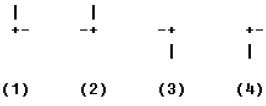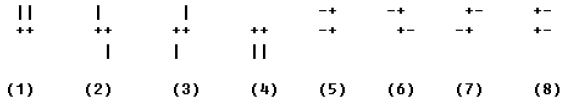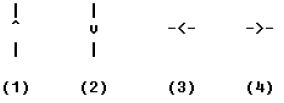Online JudgeProblem SetAuthorsOnline ContestsUser
Web Board
F.A.Qs
Statistical Charts
Problems
Submit Problem
Online Status
Prob.ID:
Register
Authors ranklist
Current Contest
Past Contests
Scheduled Contests
Award Contest
Register
Language:
F1 Circuit Design
 Time Limit: 2000MS Memory Limit: 65536K Total Submissions: 479 Accepted: 62

Description

We all know that there is an exciting F1 circuit in Shanghai, but how many of you know how to design an F1 circuit? Designing an F1 circuit is a very complex task. For example, you should know how much time the best F1 driver will take to finish a lap. In this task, we will ask you to solve this problem.

An F1 circuit consists of straight raceways, turns and a starting point. We use '-' (with ASCII number 45) or '|' (with ASCII number 124) to describe a section of straight raceways, and use '+' (with ASCII number 43) to describe a turn. If two straight raceways connect to a turn, we call the turn a simple turn, and the speed of an F1 race car entering and leaving a simple turn should not exceed 45 m/s. If two turns are connected with each other, this is a composite turn, and the speed of an F1 racing car entering and leaving a composite turn should not exceed 20 m/s.

A simple turn is in one of the four formats shown in Figure 1:Figure 1 Formats of simple turns

A composite turn is in one of the eight formats shown in Figure 2:Figure 2 Formats of composite turns

You may assume that in an F1 circuit there are only the two types of turns we mentioned above.

The starting point of an F1 circuit is the beginning and ending position of an F1 race car. A starting point is in one of the four formats shown in Figure 3, meaning that the start direction is going up ('^' with ASCII number 94), down ('v' with ASCII number 118), left ('<' with ASCII number 60) or right ('>' with ASCII number 62) respectively:Figure 3 Formats of starting point

The straight raceways, turns and a starting point mentioned above will compose a closed F1 circuit.

We know that a section of straight raceway is M meters long, which indicates that a '-' or '|' equals to M meters. To make the problem easier, we ignore the length of turns and the start point. The F1 race car enters and leaves a turn with the same speed.

An F1 driver will run 3 laps in each circuit. On the first lap, the initial speed of the car leaving the starting point should be zero. There is no restriction for the second lap. On the last lap, the speed of the car arriving at the start point should also be zero. We record the running time of the second lap as the best lap time. What you need to do is to calculate the minimum best lap time.

What you may also know is that the acceleration of an F1 car doesn't exceed 10 m/s2, and the deceleration doesn't exceed 30 m/s2.

Input

There are several test cases. The first line of each test case contains an integer M (1 <= M <= 200), which is the length of a section of straight raceways. The next several lines will contain a map of an F1 circuit. A circuit map only contains the circuit, some white spaces and some blank lines. The description will occupy 50 lines or less, and the number of characters in each line will not exceed 100. A test case with M = 0 ends the input, and should not be processed.

Output

For each test case, output a line containing the best loop time in seconds. You should round the result to two digits after the decimal point.

Sample Input

```199
+-------------+
+----+        |
+----+++      ^
|     ||      |
|     ||      |
+-----++------+
0
```

Sample Output

`97.34`

Source

[Submit]   [Go Back]   [Status]   [Discuss]Home PageGo BackTo top